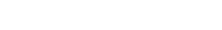# Jianhong Xu | Mathematics | SIU

HomeCONTACT

SIU.EDU

## Jianhong Xu

### ProfessorP: 618.453.6561
E: jhxu@siu.edu
O: Neckers 387

http://neumann.math.siu.edu

Professor; Ph.D., University of Connecticut, 2003. Numerical analysis, matrix computations, matrix theory and applications.

## Research Interests

Numerical analysis, matrix computations, parallel algorithms, matrix theory and applications.

## Selected Publications

1. Generalized $\lambda$-Newton inequalities revisited, Journal of Inequalities in Pure and Applied Mathematics, 10 (2009), Art. 19.
2. Markov chain small-world model with asymmetric transition probabilities, Electronic Journal of Linear Algebra, 17 (2008), 616-636.
3. Transition matrices for well-conditioned Markov chains, Linear Algebra and Its Applications, 424 (2007), 118-131 (with S. Kirkland and M. Neumann).
4. A note on Newton and Newton-like inequalities for M-matrices and for Drazin inverses of M-Matrices, Electronic Journal of Linear Algebra, 15 (2006), 314-328 (with M. Neumann).
5. Matrix analysis of a Markov chain small-world model, Linear Algebra and Its Applications, 409 (2005), 126-146 (with M. Catral and M. Neumann).
6. Convexity and elasticity of the growth rate in size-classified population models, SIAM Journal on Matrix Analysis and Applications, 26 (2004), 170-185 (with S. Kirkland and M. Neumann).